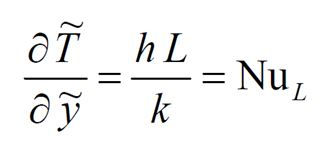top of page
Search
• manchestercfd

# What you need to know about ‘Heat Transfer’ in CFD

1. Modes of Heat Transfer

Conduction, convection and radiation are the three mechanisms by which thermal energy may be transferred from one point in space (and time) to another.

• Conduction: diffusion of heat due to temperature gradients. A measure of the amount of conduction for a given gradient is the heat conductivity.

• Convection: when heat is carried away by moving fluid. The flow can either be caused by external influences, forced convection; or by buoyancy forces, natural convection. Convective heat transfer is tightly coupled to the fluid flow solution.

• Radiation: transfer of energy by electromagnetic waves between surfaces with different temperatures, separated by a medium that is at least partially transparent to the (infrared) radiation. Radiation is especially important at high temperatures, e.g. during combustion processes, but can also have a measurable effect at room temperatures.2. Overview of Dimensionless Numbers

Nusselt Number

The Nusselt number (Nu) represents the relative magnitude of “real” heat flux to conduction heat flux and is essentially a dimensionless heat transfer coefficient.

The Nusselt number is derived through equating the heat conducted from the wall to the same heat transfer in convective terms:(92)

where k is the thermal conductivity and h is the convective heat transfer coefficient.

Then by defining the following dimensionless quantities:The definition of Nusselt number is obtained through:Therefore, the Nusselt number is a dimensionless ratio of convective to conductive heat transfer.

Prandtl Number

Prandtl number is the ratio between momentum diffusivity and thermal diffusivity and is defined as:Typical values are Pr = 0.01 for liquid metals; Pr = 0.7 for most gases; Pr = 6 for water at room temperature.

Grashof Number

Grashof number (Gr) is the ratio between buoyancy forces and viscous forces:Rayleigh Number

Natural convection problems are characterized using the Rayleigh number. The Rayleigh number governs natural convection phenomena (Ra = Gr.Pr):Reynolds Number

The Reynolds Number (Re) is the ratio between inertial and viscous forces and is defined as:Froude Number

In most industrial applications, free and forced convection occur simultaneously. The relative magnitude of these effects can be determined by using a modified Froude number, Fr.

In the definition of the above dimensionless numbers:

b is the thermal expansion coefficient, g is the acceleration to gravity, ν is the kinematic viscosity (=μ/ρ), α is the thermal diffusivity (=k/ρcp), L is the length-scale and T is temperature.

bottom of page